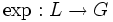# Lazard correspondence establishes a 1-isomorphism between Lazard Lie group and Lazard Lie ring

(diff) ← Older revision | Latest revision (diff) | Newer revision → (diff)

## Statement

Suppose$G$ is a Lazard Lie group and$L$ is its Lazard Lie ring with$\log:G \to L$ the logarithm map and$\exp:L \to G$ the exponential map. (These are both bijections, and are inverses of each other).

Then,$\log$ and$\exp$ are 1-isomorphisms, i.e., they are isomorphisms when restricted to cyclic subgroups.

## Facts used

1. Logarithm map from Lazard Lie group to its Lazard Lie ring is a quasihomomorphism

## Proof

### Proof outline

This follows directly from fact (1), and the fact that the logarithm map is bijective.